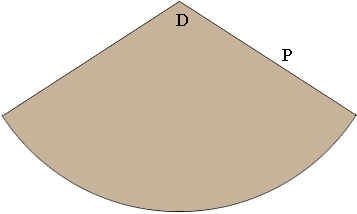SEARCH HOMEMath Central Quandaries & QueriesQuestion from Izzy: Hello, I need to create a cone as a prop and my math is not good enough to create the pattern. Here are my instructions : The diameter is 2'5" and it has to be 4 feet tall. It is a giant cone. I want it to be pointy at the end, not truncated. Thanks for your help.HI,

The pattern piece is a giant slice of pie with radius P units and an angle of D degrees.Suppose the cone you want has base radius of R units and height H units, in your case that's R = 1.25 inches and H = 48 inches. Formulas for calculating D and P are contained in a response Stephen sent to a previous question.

Here's the "general" solution for any similar cone. Let's say you know the height H and the opening you want has a radius of R.

Let P = the radius of the circle. Then P = √(R² + H²).
Let D = the number of degrees of the circle to keep (as a pie slice). Then D = 360(R/P).

PennyMath Central is supported by the University of Regina and The Pacific Institute for the Mathematical Sciences.